# Expressions Equations and Inequalities Ch 1 3 Algebraic

• Slides: 12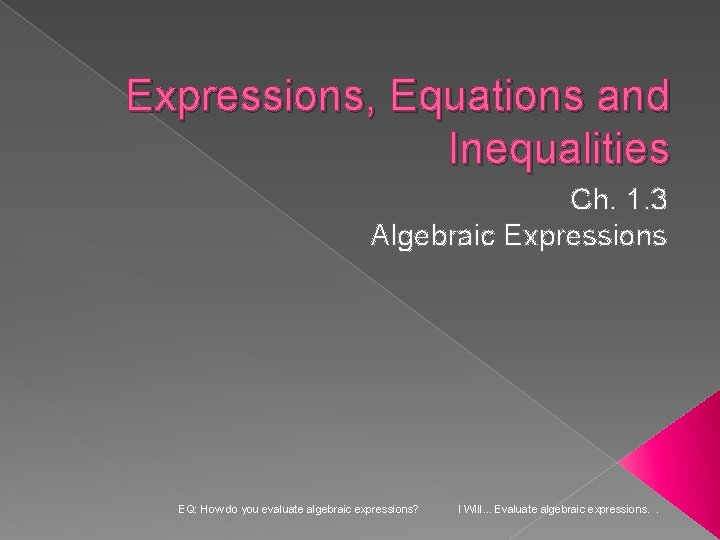Expressions, Equations and Inequalities Ch. 1. 3 Algebraic Expressions EQ: How do you evaluate algebraic expressions? I Will. . . Evaluate algebraic expressions. .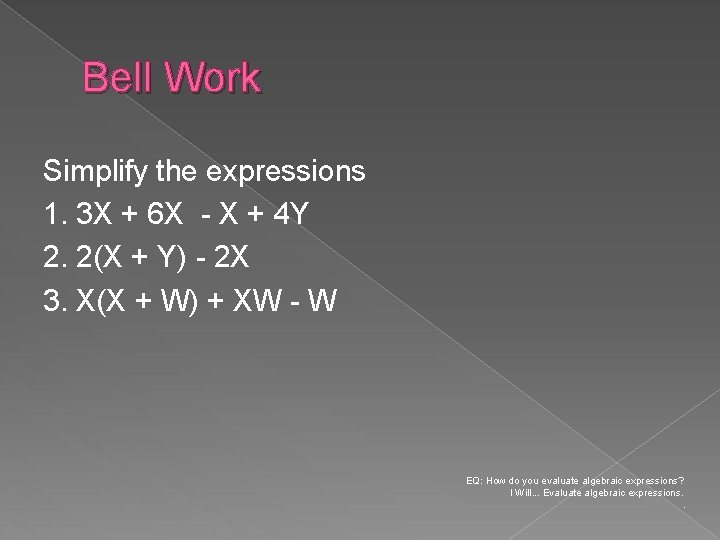Bell Work Simplify the expressions 1. 3 X + 6 X - X + 4 Y 2. 2(X + Y) - 2 X 3. X(X + W) + XW - W EQ: How do you evaluate algebraic expressions? I Will. . . Evaluate algebraic expressions. .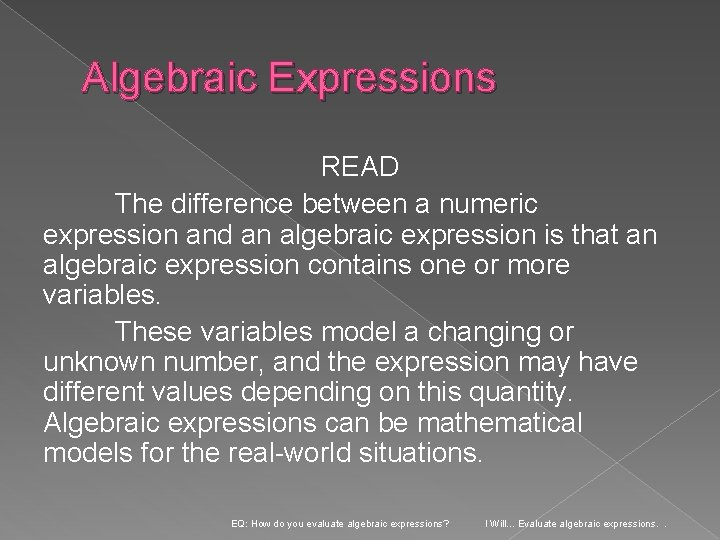Algebraic Expressions READ The difference between a numeric expression and an algebraic expression is that an algebraic expression contains one or more variables. These variables model a changing or unknown number, and the expression may have different values depending on this quantity. Algebraic expressions can be mathematical models for the real-world situations. EQ: How do you evaluate algebraic expressions? I Will. . . Evaluate algebraic expressions. .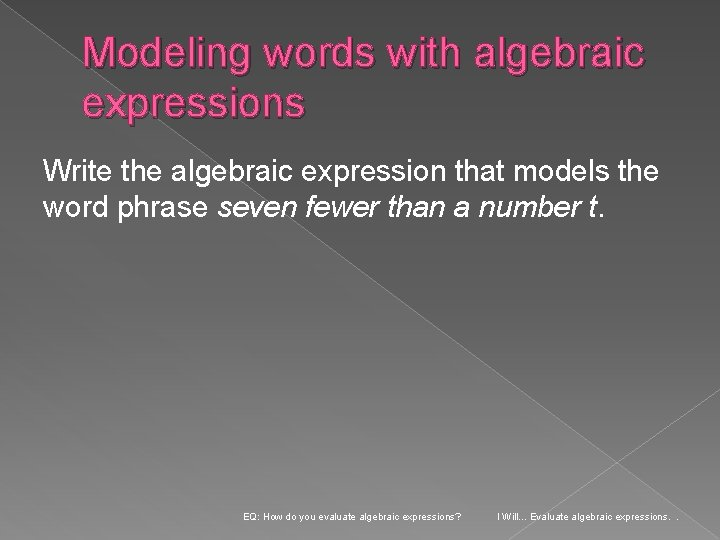Modeling words with algebraic expressions Write the algebraic expression that models the word phrase seven fewer than a number t. EQ: How do you evaluate algebraic expressions? I Will. . . Evaluate algebraic expressions. .Modeling words with algebraic expressions Now you try… 1. Write the algebraic expression for the word phrase two times the sum of a and b. 2. Write the algebraic expression for the word phrase the quotient of the difference between 5 and a number n, and 2. EQ: How do you evaluate algebraic expressions? I Will. . . Evaluate algebraic expressions. .Modeling a Situation You start with \$20 and save \$6 each week. Write an algebraic expression that models the total amount you save? EQ: How do you evaluate algebraic expressions? I Will. . . Evaluate algebraic expressions. .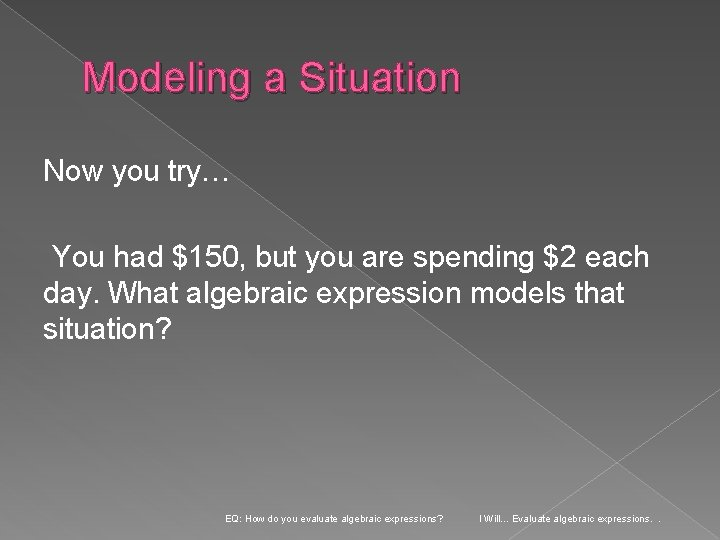Modeling a Situation Now you try… You had \$150, but you are spending \$2 each day. What algebraic expression models that situation? EQ: How do you evaluate algebraic expressions? I Will. . . Evaluate algebraic expressions. .Evaluating Algebraic Expressions What is the value of the expression for the given values of the variables? 7(a+4) + 3 b – 8 a=-4 and b= 5 EQ: How do you evaluate algebraic expressions? I Will. . . Evaluate algebraic expressions. .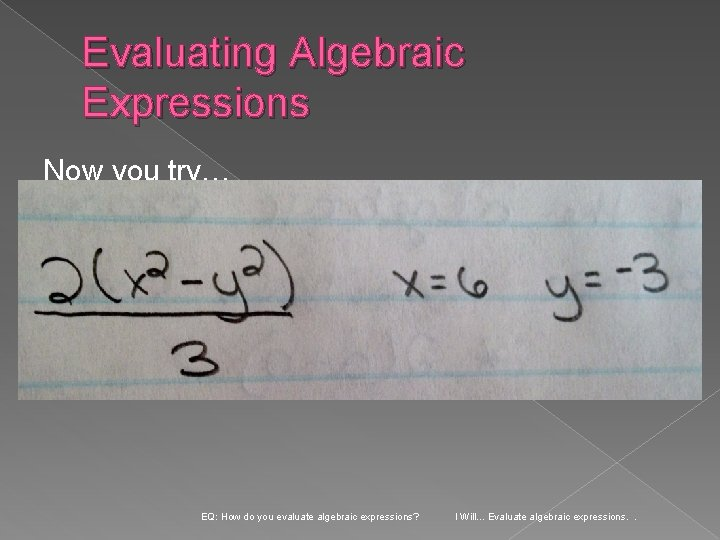Evaluating Algebraic Expressions Now you try… EQ: How do you evaluate algebraic expressions? I Will. . . Evaluate algebraic expressions. .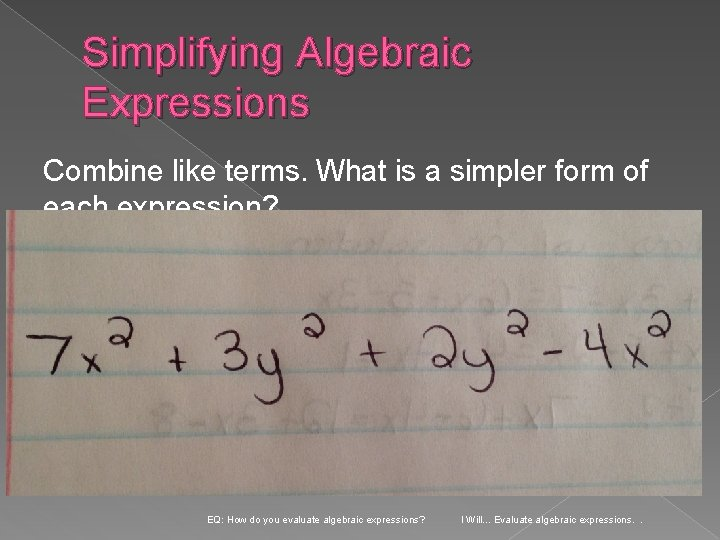Simplifying Algebraic Expressions Combine like terms. What is a simpler form of each expression? EQ: How do you evaluate algebraic expressions? I Will. . . Evaluate algebraic expressions. .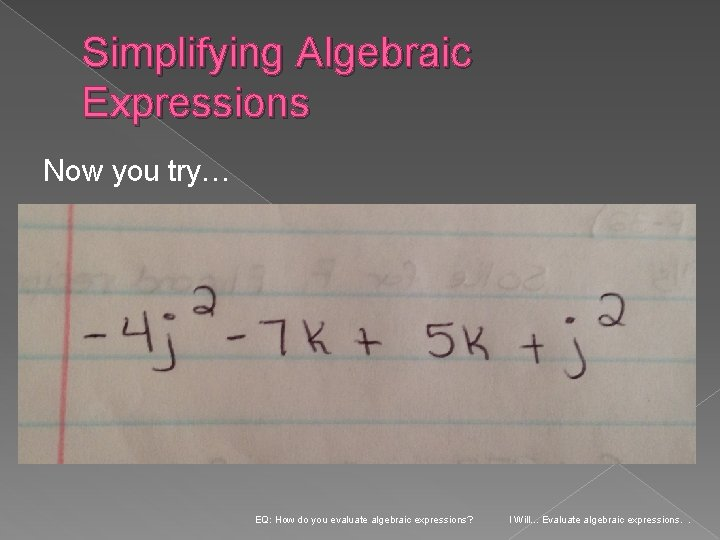Simplifying Algebraic Expressions Now you try… EQ: How do you evaluate algebraic expressions? I Will. . . Evaluate algebraic expressions. .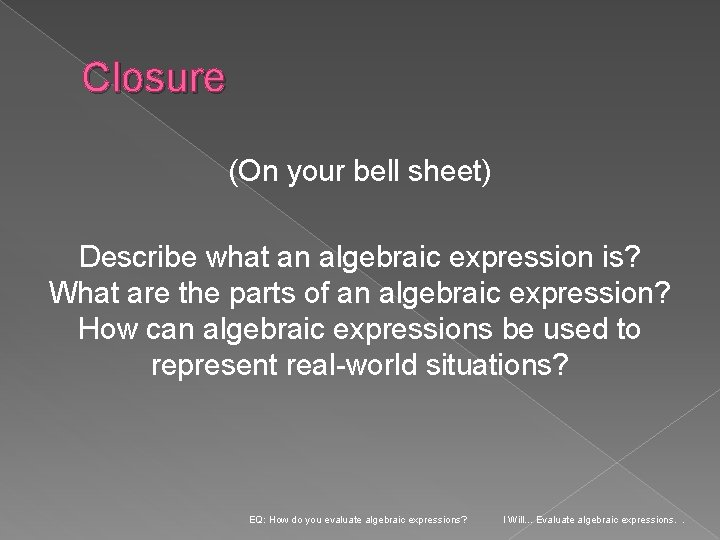Closure (On your bell sheet) Describe what an algebraic expression is? What are the parts of an algebraic expression? How can algebraic expressions be used to represent real-world situations? EQ: How do you evaluate algebraic expressions? I Will. . . Evaluate algebraic expressions. .